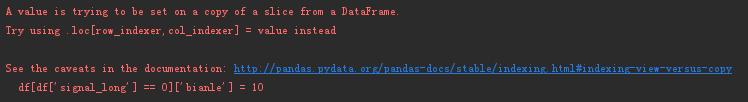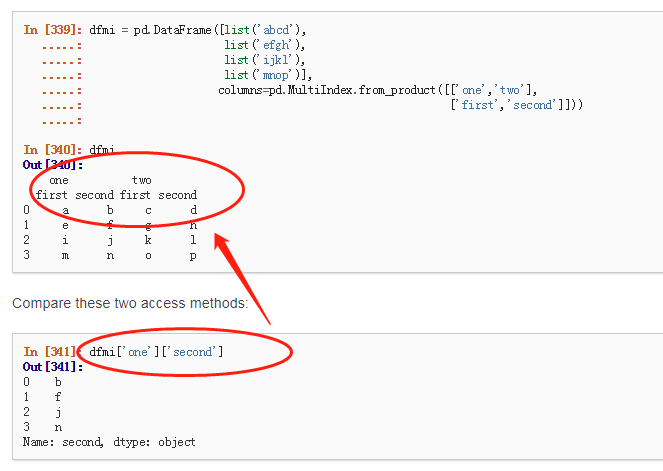• df.assign(** kwargs)#创建或修改添加到原数据中 参数： #kwargs：关键字值对(列名，【函数，系列，标量或数组】) 返回：df  2.实例1：  s=pd.Series([11,12,13],name='S') data=np.arange(21,24) df=pd....
assign添加列 2019/1/4
1.函数：
df.assign(** kwargs)#创建或修改列并添加到原数据中

参数： #kwargs：关键字值对(列名，【函数，系列，标量或数组】)
返回：df 
2.实例1：
s=pd.Series([11,12,13],name='S')
data=np.arange(21,24)
df=pd.DataFrame({'A':[31,32,33],'B':[41,42,43]})
fun=lambda x:x.A+x.B

df.assign(C=fun,D=df.A+df.B,E=s,F=data)#增加新列

A  B  C  D  E  F
0 31 41 72 72 11 21
1 32 42 74 74 12 22
2 33 43 76 76 13 23 
实例2：
df.assign(C=fun,B=data)#修改原数据
df.assign(B=data,C=fun)#修改原数据 注意顺序不同，结果不同

A  B  C    A  B  C
0 31 21 72 0 31 21 52
1 32 22 74 1 32 22 54
2 33 23 76 2 33 23 56 
实例3：
df=pd.DataFrame({'A':[31,32,33],'B':[41,42,43]})
df.assign(B=df.A, C=lambda x:x['A']+ x['B'])# 关键字参数相互依赖位置Python3.6以上版本

A  B  C
0 31 31 62
1 32 32 64
2 33 33 66
=======================================
#所有Python版本兼容的代码，请将赋值分成两部分
df = pd.DataFrame({"A": [1, 1, 1]})
(df.assign(A=lambda x: x['A'] + 1)
.assign(B=lambda x: x['A'] + 2))

A B
0 2 4
1 2 4
2 2 4 
实例4：
DataFrame添加
s1=pd.Series([11, 12, 13], index=['a', 'b', 'c'])
s2=pd.Series([21, 22, 23, 24], index=['a', 'b', 'c', 'd'])
df = pd.DataFrame({'A' : s1,'B' : s2})

#添加列
df['C']=pd.Series([40,41,42],index=['a','b','c'])#数据帧添加1列;原数据改变
df['D']=df['A']+df['C']                          #数据帧添加1列;原数据改变

# df df df
A  B      A  B    C      A  B    C D
a 11.0 21 a 11.0 21 40.0 a 11.0 21 40.0 51.0
b 12.0 22 b 12.0 22 41.0 b 12.0 22 41.0 53.0
c 13.0 23 c 13.0 23 42.0 c 13.0 23 42.0 55.0
d NaN  24 d NaN  24 NaN  d NaN  24 NaN NaN 

展开全文• 在数据处理过程中,经常要对符合某种条件的行列进行操作,有时候会犯迷糊,这里详细解释一下pandas是怎么识别df[]的. df[&lt;条件&gt;] # 得到的是一个新的df1,对其进行任何操作,对原来的df不产生影响. type(df...
在数据处理过程中,经常要对符合某种条件的行列进行操作,有时候会犯迷糊,这里详细解释一下pandas是怎么识别df[]的.
df[<条件>]   # 得到的是一个新的df1,对其进行任何操作,对原来的df不产生影响.
type(df[<条件>])   # 数据类型是DataFrame
>> <class 'pandas.core.frame.DataFrame'>

df['列名']   # 得到的是df中'列名'这一列,
type(df['列名'])   # 数据类型是series
>> <class 'pandas.core.series.Series'>

df['新列名'] = 0   # 原df发生改变,在df中添加新列,且新列取值为0

df[<条件>]['新增列名']=0
# 这么写的本意是获取满足条件的新的df1,然后对新的df1增加新的一列,并将新的一列赋值为0,但此时会报错
# df[][] 这种结构,python会自动识别为获取Multiindex,具体见下图

=======================================================
=======================================================
df.loc[<条件>][<新增列名>]
# 本质是一个函数.loc[row_index,col_index]有自己特定的语法规范
# df.loc[<条件>][<新增列名>]是想当然的,是不合语法的

当输入,
df[<条件>]['新增列名']=0会出现上图提醒,并给出参考链接,意思是df[][]默认是寻求Multiindex多级索引.此时不得不感慨一下Python的强大,其实此处Python直接提醒一下index错误就可以了,但是她没有偷懒,而是明白了你的意思,给你一个参考解决方案.
.
.
.
2018-11-26 18:48:39写于滨州市太阳花咖啡书屋
展开全文Multiindex
• pandas -V 0.25.3 numpy -V 1.17.4 python -V 3.8.0 1、指定位置修改值 import numpy as np import pandas as pd # 创建pandas的DataFrame dates = pd.date_range('...df = pd.DataFrame(np.arange(24).reshape((6...
作者：小白蒋， 所有的努力都是为了做个小白，单纯且快乐~ pandas -V 0.25.3 numpy -V 1.17.4 python -V 3.8.0

本文内容
1、指定位置修改值2、根据条件修改值3、添加新列

1、指定位置修改值
import numpy as np
import pandas as pd
# 创建pandas的DataFrame
dates = pd.date_range('20191227','6')

df = pd.DataFrame(np.arange(24).reshape((6,4)),index=dates,columns=['A','B','C','D'])

print(df)

输出
             A   B   C   D
2019-12-27   0   1   2   3
2019-12-28   4   5   6   7
2019-12-29   8   9  10  11
2019-12-30  12  13  14  15
2019-12-31  16  17  18  19
2020-01-01  20  21  22  23

iloc就是根据索引定位
df.iloc[2,2] = 1111

loc就是根据标签的名称定位
df.loc['20191228','B'] = 2222

2、根据条件修改值
根据判断条件修改值
df.B[df.A>4] = 0

3、添加新列
df['F'] = np.nan

通过Series添加新列
df['E'] = pd.Series([1,2,3,4,5,6],index=pd.date_range('20191227',period=6))

展开全文pandas python
• 有默认值的添加列：alter table Test add BazaarType char(1) default(0) 删除没有默认值的：alter table Test drop COLUMN BazaarType 删除有默认值的：先删除约束（默认值）alter table Te
 添加没有默认值：alter table 表名 add BazaarType char(1)
有默认值的添加列：alter table 表名 add BazaarType char(1) default(0)
删除没有默认值的列：alter table  表名 drop COLUMN BazaarType
删除有默认值的列：先删除约束（默认值）alter table   表名 DROP CONSTRAINT DF__Test__BazaarType__3C4ACB5F，
然后在删除列alter table   表名 DROP COLUMN BazaarType

系统自带的查询约束条件的存储过程：exec sp_helpconstraint 表名
添加字段：   允许空字符: alter table 表名 add 新字段 字段类型 NULL
不允许空字符: alter table 表名 add 新字段 字段类型 not NULL
增加字段        alter table   表名 add dspcode char(200)        删除字段        ALTER TABLE   表名 DROP COLUMN 字段名        修改字段类型        ALTER TABLE   表名 ALTER COLUMN column_name new_data_type        改名        sp_rename        更改当前数据库中用户创建对象（如表、列或用户定义数据类型）的名称。
展开全文sql
• 当我们使用Python进行数据分析时，有时可能需要向DataFrame添加列，所添加要基于DataFrame的其他的值。 虽然这听起来很简单，但是，不少初学想到的是用if-else条件语句来实现，这就把问题搞复杂了。有一个简单...python 机器学习
• 选择 import pandas as pd data = {'a': [0, 1], 'b': ['x', 'y'], 'c': [5, 6]} df = pd.DataFrame(data) new_df = df[['a', 'b']] print(new_df) 删除 import pandas as pd data = {'a': [0, 1], 'b': ...
• 一、DataFrame添加列 直接通过赋值为空，添加。 >>> import pandas as pd >>> df = pd.DataFrame(np.arange(12).reshape(3, 4), index = ['row1', 'row2', 'row3'], columns=['col1', '...python 机器学习 tensorflow 深度学习
• 1. 逐行处理，当指定多的值满足一定条件时，取这几列的最大值作为该行的新值. 2. 将日期划分为上、中、下旬并作为新 代码 1.按col_night，col_day，col_whole三的值生成标志字段rs_flag，并取它们的最大...python
• 2、多列条件同时满足使用& 3、多列条件或 | 拓展学习 import pandas as pd import numpy as np df1 = pd.DataFrame(np.array([[1, 2, 3], [4, 5, 6], [7, 8, 9]]), columns=['a', 'b', 'c'],index=['i1','i...python pandas 筛选法 数据挖掘
• 添加没有默认值：alter table Test add BazaarType char(1) 有默认值的添加列：alter table Test add BazaarType char(1) default(0) 删除没有默认值的：alter table Test drop COLUMN BazaarType...sql table object null 数据库
• 以前又傻又菜的时候，根据条件替换某一值或新增总是会写一些for 循环去处理，现在发现一个更简洁的方式，就是善用pandas方法。 根据条件新增一值 现有数据集如下所示： 调用apply()方法，可以作用于Series...pandas python 数据分析
• 我想创建一个新，根据col1的index来选择col2的item， 例如：  index col1 col2 col3 1 [1,2] [apple, peer, oragne, banana] [apple,peer] 2  [pancake, soda] [soda]  请问应该如何操作？list python 数据挖掘
• pandas 根据筛选条件对指定excel进行筛选导入需要筛选的excel和筛选条件要筛选的表格A思路结果 这两天老师要求筛选一下最近几年学院发表的文章，从图书馆那要了份数据量较大的excel，将该excel分成学生文章、老师...python excel
• 将最后一每个数字前加上Depthdf["new"] =[ 'Depth % i' % i for i in df["Depth"]] df.head()python pandas DataFrame
• 比如，我想筛选出“设计井别”、“投产井别”、“目前井别”三数据都为11的数据，结果如下： 当然，这里的筛选条件可以根据用户需要自由调整，代码如下： # -*- coding: utf-8 -*- import pandas as pd #input....python
• 实例如下所示： import numpy as np import pandas as pd data = {'city': ['Beijing', 'Shanghai', 'Guangzhou', 'Shenzhen', 'Hangzhou', 'Chongqing'], 'year': [2016,2016,2015,2017,2016, 2016], ...
• import numpy as np import pandas as pd ...# 使用apply函数, 如果city字段包含'ing'关键词，则'判断'这一赋值为1,否则为0 frame['panduan'] = frame.city.apply(lambda x: 1 if 'ing' in x else 0) print(frame)
• Python中数据框的方法map(function or dict) Python中的数据框，在...比如：在df数据框中存在一名为 ‘age’，但是取值为 “中年”、“青年”、“老年”。我们想把这种字符串转换为1,2,3. data['age1']=data[...
• alter table Test add BazaarType char(1)有默认值的添加列：alter table Test add BazaarType char(1) default(0)删除没有默认值的：alter table Test drop COLUMN BazaarType删除有默认值的：先删除约束...sql table object null 数据库
• 1 引言 Pandas是作为Python数据分析著名的工具包，提供了多种数据选取的方法，方便实用。本文主要介绍Pandas的几种数据选取的方法。 Pandas中，数据主要保存为Dataframe和...　** 1）行（）选取（单维度选取）：d......